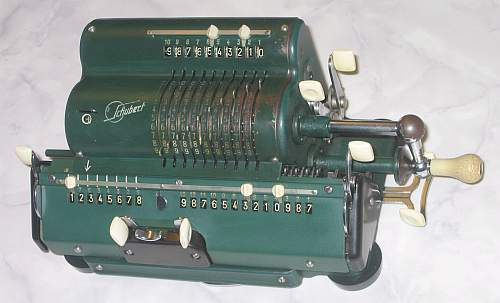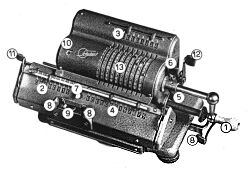previous <<==>> next

 SCHUBERT   DRVHave a look at the Restoration Procedure...
 ``` T E C H N I C A L D A T A of the Mechanical Calculator SCHUBERT ************************************************************************ Model » DRV « Serial Number: 65K284 Dimensions: (ca.) Width = 11 " / 28 cm Depth = 5_1/2" / 14 cm Height = 5 " / 13 cm Weight: (ca.) 12 lbs / 5.4 kg Mechanics: Pin-Wheel / Sprossenrad 10s Carry Mechanism Functions: Add, Subtract, Multiply, Divide Back-Transfer / Rueckuebertragung Registers: Input = 10 Decimals Counter = 8 Decimals Arithmetic = 13 Decimals Manufacturer: SCHUBERT Rastatt / Baden Germany 1956 H O W T O U S E the SCHUBERT »DRV« **************************************** ( Refer to the Picture from the Manual ) `````` OPERATING CONTROLS 1 Crank 5 Reset 9 Move Carriage 2 Counter Arithmetic & Counter continuously 3 Input 6 Reset Input 10 Counter Direction 4 Arithmetic 7 Disable Counter-Reset 11 Reset Counter 8 Move Carriage 12 Back-Transfer Lever One Step Right or Left BASIC SETTINGS: =============== (a) Crank: ---------- For addition (+) the crank is turned clockwise; for subtraction (-) the crank is turned counter-clockwise. In the idle position the crank is DOWN & LOCKED. To make one or more turns with the crank, pull out the handle to unlock. When finished the turn(s), let the handle snap into lock again. The locked down position only makes other functions (ex. clearing) accessible. REMARK: Every started turn has to be finished completely! Accidentally started turns are correctible somehow. (b) Shifting the Carriage: -------------------------- The left position "1" is the "Start Position" of the carriage. (c) Counting Direction: ----------------------- It depends of the 1st turn of the crank how the counter is switched to (+) or (-). The counter will increase its content for positive (clock- wise) or negative (counter-clockwise) turns. When the direction of the turns is changed, the counter will decrease its content. In both cases the counter is working with 10s-carry - and allows "Shortened Method of Multiplication". (d) Clear Counter & Arithmetic: ------------------------------- Lever  clears counter & arithmetic unit - Lever  counter only. ( When Key  is pressed, Lever  clears arithmetic unit only ) (e) Back Transfer: ------------------ For continuous multiplication the contents of the arithmetic unit can be back transfered into the input register with this procedure: Push & HOLD lever ; Pull lever , release [6 & 12]; Pull  A ==>> I ; A & C cleared ADDITION & SUBTRACTION: ======================= Example: 123 + 45 - 6 = 162 Clear input, counter and arithmetic units. ADD: Enter the first number (123) in the far right of the input unit. Make a positive (clockwise) turn with the crank to transfer the number into arithmetic unit. The counting unit displays the figure 1. Enter the second number (45). Make a positive (clockwise) turn with the crank to add the number. The arithmetic unit displays the intermediate sum (168) and the counting unit displays the figure 2. SUBTRACT: Enter the third number (6). Make a negative (counter-clockwise) turn with the crank. The arithmetic unit displays the result (162) and the counting unit is decreased by 1. REMARK: NEGATIVE RESULTS are displayed in the arithmetic unit as the COMPLEMENT of the next higher 10, 100, 1000, ... Example: -12 = 99...9988 MULTIPLICATION: =============== Example: 123 x 45 = 5535 Clear input, counter and arithmetic units. Enter the multiplicand (123) in the far right of the input unit. The multiplicator (45) has two digits, so the carriage is shifted to position 2. Make positive (clockwise) turns with the crank, until the first figure of the multiplicator (4) will appear in the 2nd position of the counter unit. Shift the carriage to position 1. Repeat making positive turns with the crank, until the second figure of the multiplicator (5) appears in the 1st position of the counter unit. The multiplication is done: The multiplicand (123) stays in the input unit, the multiplicator (45) in the counter and the result (5535) is in the arithmetic unit. DIVISION: ========= Example: 22 : 7 = 3.1428571 Remainder 3 Division requires 3 steps: (1st) To Set the Dividend into Arithmetic Unit: ----------------------------------------------- For the maximum number of decimals, pull out the carriage to the far right position. Enter the dividend (22) in the far right of the input unit. Make a positive (clockwise) turn with the crank to transfer into arithmetic unit. (2nd) To Set the Divisor into Input Unit: ----------------------------------------- Clear counter & input units. Enter the divisor (7) above the dividend (22). (3rd) To Divide: ---------------- Make negative (counter-clockwise) turns with the crank until the arithmetic unit shows an "underflow". Make one positive (clockwise) turn with the crank. Move the carriage to the next left position. Repeat this procedure until the required number of decimals ... The result (3.1428571) is in the counter unit, and the remainder (3) is in the arithmetic unit. The divisor (7) stays in the input unit, therefore an additional decimal can be estimated... ( 4, cause 4 x 7 = 28). Have a look at "Calculating Trickies" ... R E M A R K S : ======================================================================== (1st) There is a "Transport Lock" on the left side of the carriage. Make sure that the SCREW ( from bottom side ) is released. (2nd) Until 1958 the first two figures of the serial number exchanged give the year of production ==>>> SN = 65K284 was made in 1956 impressum: ************************************************************************* © C.HAMANN http://public.BHT-Berlin.de/hamann 11/06/08 ```